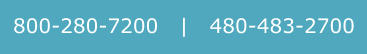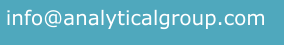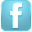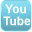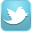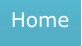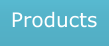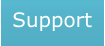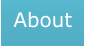## WinCross Frequently Asked Questions (FAQs)APPLICATION LIMITS

1. What is the logic limit in WinCross?

1024 characters is the limit when entering logic in a banner, filter or row.

2. What if my logic requires more than 1024 characters?

Use the ASSIGN statement in the Glossary to create new variables.

3. How many rows can be displayed on a single table?

6,000.

4. What are the record/file size limits in WinCross?

Limit of 65,536 characters and 32,768 variables.

5. How many banner columns can I have?

You can have a maximum of 255 banner columns per banner.

6. What are “reserved” WinCross variable names?

WinCross allows for the use of variable names. However, some variable names are ineligible in that they are reserved for the exclusive use of WinCross.

Please see the WinCross help topic Reserved Key Words for full details.

GENERAL

1. What are the rules for rounding values in WinCross?

You can specify how you want WinCross to round percents and statistics in Setup|Job Settings|Rounding.

There are two options for rounding. The first option is to round up values over 5 and round down values under 5. For rounding equal to 5, round to the nearest even number. The second option is to always round up values of 5 or more and round down values less than 5.

Rounding in WinCross is based upon three general rules:

Rule 1 - If the remainder beyond the last digit to be reported is less than 5, drop the last digit. Rounding to one decimal place, the number 5.3467 becomes 5.3.

Rule 2 - If the remainder is greater than 5, increase the final digit by 1. The number 5.798 becomes 5.8 if rounding to 1 digit.

Rule 3 - To prevent rounding bias, if the remainder is exactly 5, then round the last digit to the closest even number. Thus the number 3.55 (rounded to 1 digit) would be 3.6 (rounding up) and the number 6.450 would round to 6.4 (rounding down) if rounding to 1 decimal. When the number to the left of the 5 is even, no rounding occurs. For example, 4.225 would become 4.22. When the number to the left of 5 is odd, rounding will occur. For example, 4.215 would become 4.22.

See page 12 in Hurlburt, R. (1994) Comprehending Behavioral Statistics, Brooks/Cole, Pacific Grove, CA.

2. How can I look up an interview respondent who may have provided "dirty" data?

You can use the TEST statement in the Glossary to search for respondents that break certain conditions.

3. How can I select specific tables to run?

Depress and hold the Ctrl key while individually clicking on those tables you want to run, or use the Edit Selections option on the Run|Tables dialog.

4. Why doesn't WinCross recognize my variable names?

Check these three items:

A. Your data file isn’t open
B. You don’t have variable names in the first line of your data file

5. What is the difference between a table mean and row mean?

The difference between a table mean and row mean is based on the type of table you’re creating. A table mean is used where every row in the calculation of the mean has only one value. If the rows represent value ranges, then the # (pound symbol) operator must be used to give the row a single value for calculations.

A row mean is appropriate when rows represent a value range and the mean is to be calculated off the actual distribution of data. In that event, a row is created with the appropriate text and logic showing the value range you want to use. In the Row Options dialog box (Setup|Tables|Row Options) you should select the appropriate statistics (Mean, Standard Deviation, Standard Error, etc.).

6. When would I want to use indexing?

Please see the WinCross help topic Indexed Variables Examples for full details.

7. How can I write output to Excel?

WinCross tables can be written to Excel. When Run|Tables|Excel Options is selected, WinCross creates an Excel file in addition to the WinCross output file. You also have the option to save your crosstab report using File|Save|Save Report As. Note: Saving to Excel using File|Save|Save Report As produces an image of your WinCross tables and does not contain the actual values the way the Excel report from Run|Tables|Excel Options does.

Other considerations in Run|Tables:

Make sure you have also selected the Create Tables option.
Choose the directory where you want WinCross to write the Excel file(s).

8. How is weighting handled in WinCross?

WinCross has the ability to weight your crosstabs. This is done using Setup|Banners|Edit Banner|Weights and must be done after banner creation.

Weights can be created in three ways:

A. Weight variables can be part of your original data file
B. You can use glossary statements to create weighted variables
C. You can specify an actual value for a weight when you set up banners

Weights can be specified with an actual weight value, with the card/column location that holds the weight variable or the variable name for variable data. An actual weight can have up to 12 digits, can be positive or negative and can have decimal places.

9. How do I create a summary table using SPSS data?

Mean Summary tables are easily created using the Summary of Means option in Setup|Express Tables for SPSS Data. You select the group of variables, choose the code value or range of code values for the rows and WinCross creates the table for you. The Question Title from each variable selected becomes the row text for each row and the Code Value(s) or range of Code Values specified becomes the row logic.

Top/Bottom Box Summary tables can also be easily created using the Summary of Frequencies option in Setup|Express Tables for SPSS Data. You select the group of variables, choose the code value or range of code values for the rows and the code value or range of code values for the percentaging base of each row and WinCross creates the table for you. The Question Title from each variable selected becomes the row text for each row and the Code Value(s) or range of Code Values specified becomes the row logic. WinCross automatically creates two sets of rows, one set to be used for percentaging bases with the Hide row (HR) option specified and the second set of rows is automatically created with the percentaging base specified

10. How do I create a summary table using ASCII or non-SPSS variable data?

Please see the WinCross help topic Mean Summary Tables for full details.

11. Which statistics are referred to by the term "Central Tendency?"

Mean, Median and Mode.

12. Which statistics are referred to by the term "Variability?"

Standard Deviation and Standard Error.

STATISTICAL TESTING

1. What are the critical values for the confidence levels used for statistical testing?

The critical values for confidence levels are contained in this Excel document.

2. When doing significance testing table lookups, what type of test does WinCross use?

WinCross significance testing is performed using two-tailed tests.

3. Although I have statistics set up to run in my banner, they are not appearing on my tables. What am I doing wrong?

You must select Means/Percents/Chi-Square for your table statistics (Setup|Tables|Statistics) as well as for your banner.

4. How is SPSS significance testing with weighted means biased?

This article details the bias inherent in SPSS significance testing with weighted means.

5. When working with weighted data, does WinCross use the weighted sample size for statistical testing?

a. WinCross uses the Effective Sample Size if replicates are not being used (the WinCross default is not to use replicates).
b. WinCross uses the weighted sample size if replicates are being used (SPSS uses replicates).

6. What special considerations are there for volumetric Z-test for proportions?

7. What special considerations are there for the Dependent Paired/Overlap (Multi) Z-test (Part-Whole Comparisons)?

8. Why don’t my statistical tests appear on my tables?

There are two possible causes:

A. The option to include statistical testing has been added at the banner level, but has not been selected at the table level.

• Choose Setup|Tables, then select the table to which you want to add statistical testing. Next, choose Statistics, followed by the preferred tests to run. This process only adds statistical testing to the selected table (as opposed to the banner).
• Another way to globally add this option to multiple tables is to modify the table statistics. First, choose Setup|Globally Modify Tables|Table Statistics. Choose Means, Percents and/or Chi-Square. Next, select the tables to apply this to (or choose Select All), then choose the Add to existing settings option.

B. Statistical testing has been selected at the table level but not the banner level, or there are no comparison groups in the banner.

• Make sure statistical testing has been selected at the banner level. You cannot choose statistical testing for a banner until comparison groups have been added.
• WinCross requires that statistical testing be selected at both the table level AND the banner level.

9. How do I interpret the One-way ANOVA column indicators on my WinCross table?

One-way ANOVA tests are performed on columns of data within each specified WinCross comparison group. WinCross will assign a #1 to the first subset of data within each comparison group where no significant differences were found. Likewise, WinCross will assign a #2 to the next subset of data within the same comparison group where no significant differences were found. This process continues until all subsets of data have been examined for each comparison group.

A column of data can be a member of multiple subsets of data where no significant differences were found. In this case, you will see multiple One-way ANOVA group indicators under that column of the banner on the mean row of your WinCross report. For example, #1#2#3 under a column of data indicates this value is a member of subset 1, subset 2 and subset 3 for that comparison group.

10. Which WinCross statistical/significance test should I use?

11. What is the "design effect" / effective sample size in WinCross?

SAMPLE BALANCING

1. What is Sample Balancing? Is it the same as rim weighting? Are negative weights possible?

SAMPLE SIZES

1. How do I determine sample sizes for monadic and paired comparison studies?

FIELD WIDTH ERROR

1. Why do I get a "Field width does not match code values" error?

There is a value wider than the programmed logic. For example, logic of the form Q2 (1-99) or 1/5:2 (1-99) is in use, but a value larger than 99 was encountered in the data.

MICROSOFT SURFACE RT

1. Does WinCross run on Microsoft Surface RT tablet computers?

WinCross is not compatible with the Microsoft Surface RT tablet, though it will run on versions of the Surface that include an option to run a genuine Windows operating system. The Surface 3 tablet is one that provides an option to run genuine Windows.

The following documents cover various topics relating to weighted significance testing, and to part-whole comparison of means:

Weighted Data and Significance Testing Tools

Weighted Standard Error and its Impact on Significance Testing (WinCross vs. Quantum & SPSS)

Alternative Approaches to Significance Testing with Weighted Means

A Simulation Comparison of WinCross, SPSS, and Mentor Procedures for Estimating the Variance of a Weighted Mean

An Analysis of WinCross, SPSS, and Mentor Procedures for Estimating the Variance of a Weighted Mean

Part-Whole Comparison of Means

A Note on Spurious Significance

Statistical Inference about Net Promoter Scores